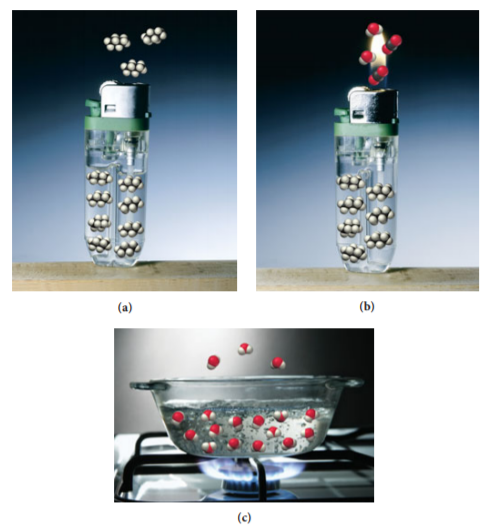×
Get Full Access to Chemistry: A Molecular Approach - 3 Edition - Chapter 1 - Problem 50e
Get Full Access to Chemistry: A Molecular Approach - 3 Edition - Chapter 1 - Problem 50e

×

# Solved: Based on the molecular diagram, classify eachISBN: 9780321809247 1

## Solution for problem 50E Chapter 1

Chemistry: A Molecular Approach | 3rd Edition

• Textbook Solutions
• 2901 Step-by-step solutions solved by professors and subject experts
• Get 24/7 help from StudySoup virtual teaching assistantsChemistry: A Molecular Approach | 3rd Edition

4 5 1 408 Reviews
30
0
Problem 50E

Problem 50

Based on the molecular diagram, classify each change as physical or chemical.Step-by-Step Solution:
Step 1 of 3

Solution 50E: All digits of a measurement including uncertain number are called significant figures. Significant figures = all certain digits + one uncertain digit There are two types of number in scientific work. (i) exact - exactly the values are known when it is measured. The values which are obtained from any measurement is completely certain value (ii) Inexact number- uncertainty in the numbers. The measured values are uncertain. The distance from Grand Rapids, Michigan, to Detroit is listed in a road atlas as 153 miles. Distance is always used to measure by using instrument.So here, distance is not an exact number. This is because, distance measurement is always uncertain and it is due to the instrumental error. Secondly, different people will make the difference to measure the distance in same measurement. This is called Human error. So the distance from Grand Rapids, Michigan, to Detroit is listed in a road atlas as 153 miles is always an inexact number due to its human and instrumental error. Precision is generally used to measure the uncertainties in the measured values. So it defines that how closely the individual measurement matches with each other. To make it confirm about the high degree of precision, identify their significant figures and measured the value in several times. Thus, when the significant figures are greater, it implies higher degree of precision of the measurement. The value of precision is measured by using the standard deviation. It confirms the difference in values from the average to individual measurement. Therefore, the value obtain from standard deviation (average value) is more precise than the value of actual measurement.

Step 2 of 3

Step 3 of 3

##### ISBN: 9780321809247

This textbook survival guide was created for the textbook: Chemistry: A Molecular Approach, edition: 3. The full step-by-step solution to problem: 50E from chapter: 1 was answered by , our top Chemistry solution expert on 02/22/17, 04:35PM. The answer to “on the molecular diagram, classify each change as physical or chemical.” is broken down into a number of easy to follow steps, and 11 words. This full solution covers the following key subjects: analysis, atlas, contribute, describe, Detroit. This expansive textbook survival guide covers 82 chapters, and 9454 solutions. Chemistry: A Molecular Approach was written by and is associated to the ISBN: 9780321809247. Since the solution to 50E from 1 chapter was answered, more than 1655 students have viewed the full step-by-step answer.

Unlock Textbook Solution